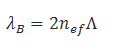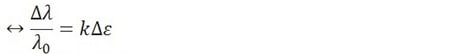A fiber Bragg grating (FBG) is a microstructure typically a few millimeters in length that can be photo inscribed in the core of a single mode fiber. This is done by transversely illuminating the fiber with a UV laser beam and using a phase mask to generate an interference pattern in its core. This will induce a permanent change in the physical characteristics of the silica matrix (fig. 1). This change consists in a spatial periodic modulation of the core index of refraction that creates a resonant structure.

Protected with a primary coating, the diameter of the fiber is 250 micrometers. Without this coating, the fiber has a diameter of 125 micrometers. The light then travels within the core, which has a diameter of approximately 8 micrometers.

## Benefits

### Operation

As a resonant structure, the FBG will act as a wavelength selective mirror; it is a narrow band filter. This means that if light from a broadband source is injected in the optical fiber, only light within a very narrow spectral width centered at the Bragg wavelength will be back-reflected by the grating. The remaining light will continue through the optical fiber to the next Bragg grating without experiencing any loss (fig. 2).

The Bragg wavelength is defined by the period of the microstructure and the index of refraction of the core.

The FBG is a symmetric structure, so it will always reflect light at the Bragg wavelength no matter which side the light is coming from.

### Acting as a strain sensor

An FBG has unique characteristics to perform as a sensor. For example, when the fiber is stretched or compressed, the FBG will measure strain. This happens because the deformation of the optical fiber leads to a change in the period of the microstructure and of the Bragg wavelength (fig. 3).

### Acting as a temperature sensor

Sensitivity to temperature is also intrinsic to a fiber Bragg grating. In this case, the main contributor to Bragg wavelength change is the variation of the silica refraction index induced by the thermo-optic effect (fig. 4). There is also a contribution from the thermal expansion which alters the period of the microstructure. However, this effect is marginal given the low coefficient of thermal expansion of silica.

### Multiplexing

One of the main advantages of this technology is its intrinsic multiplexing capability. In fact, hundreds of fiber Bragg gratings can be written on a single optical fiber, which can be as close as a few millimeters or separated by a few kilometers (fig. 5). With proper packaging, each of these microstructures can be made sensitive to parameters other than temperature or strain. For example, pressure, acceleration, displacement, etc., grants the array of sensors a multifunctional characteristic. It is important to emphasize that all the sensors can be addressed using a single optical source. Also, the addition of more and more sensors on the same fiber results in only minor loss and no crosstalk, as long as enough spectral band of the spectrum of light is reserved for each sensor (fig. 6).

### Benefits

Being a fiber optic sensor, a Bragg grating has all the advantages usually attributed to these devices, such as low loss relative to the fiber length, immunity to electromagnetic and radio frequency interference, small size and weight, intrinsically safe operation in environments characterized by hazardous materials, high sensitivity and long-term reliability. In addition, fiber Bragg grating technology reveals an inherent serial multiplexing capacity and an ability to provide absolute measurements without the need for referencing. This makes it the natural alternative to conventional electrical sensing technologies.

### Related Products

newLight | Optical Fiber Sensors
Optical sensors for demanding testing and monitoring applications. Combine them with HBM's optical interrogators for a complete measurement chain.

To top

## What are the measuring principles of a fiber Bragg grating?

The Bragg wavelength (λB) is essentially defined by the period of the microstructure (Λ) and the index of refraction of the core (nef).Equation 1

A fiber Bragg grating has unique characteristics to perform as a sensor. For example, when the fiber is stretched or compressed, the FBG will measure strain. This happens essentially because the deformation of the optical fiber leads to a change in the period of the microstructure and, consequently, of the Bragg wavelength. There is also some contribution from the variation of the index of refraction, through the photoelastic effect.

Sensitivity to temperature is also intrinsic to a fiber Bragg grating. In this case, the main contributor to Bragg wavelength change is the variation of the silica refraction index, induced by the thermo-optic effect. There is also a contribution from the thermal expansion, which alters the period of the microstructure. This effect is, however, marginal given the low coefficient of thermal expansion of silica.

### Fiber Bragg Grating (FBG) strain dependence

The strain dependence of a fiber Bragg grating can be determined by differentiating the wavelength:Equation 2

Where:

k – k factor of the Bragg grating
pe – photoelastic constant (variation of index of refraction with axial tension)

The pe for the optical fiber isMeaning that strain Sensitivity of a FBG is given by the expression:Equation 3

For FBG @1550 nm (typical) we can simplify its strain sensitivity to:Equation 4

### Fiber Bragg Grating (FBG) temperature dependence

Similarly to the strain dependence of a fiber Bragg grating, the temperature dependence can be determined by differentiating the wavelength expression (Equation 1):Equation 5

Where:
α – coefficient of thermal expansion of the fiber
ζ– thermo-optic coefficient (dependence of the index of refraction on temperature)

For a temperature sensitivity approximation, we can assume that these values are constant for the temperature range:
α= 0.55x10-6/ºC
ζ= 5.77 x10-6/ºC

Meaning that the approximate thermal sensitivity is given byEquation 6

For a typical FBG @ 1550nm we can simplify its temperature sensitivity to:Equation 7

To top